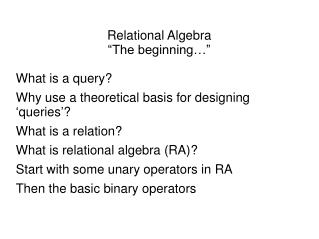DownloadDownload PresentationRelational Algebra “ The beginning… ” What is a query?

# Relational Algebra “ The beginning… ” What is a query?

Download Presentation## Relational Algebra “ The beginning… ” What is a query?

- - - - - - - - - - - - - - - - - - - - - - - - - - - E N D - - - - - - - - - - - - - - - - - - - - - - - - - - -
##### Presentation Transcript

1. Relational Algebra “The beginning…” What is a query? Why use a theoretical basis for designing ‘queries’? What is a relation? What is relational algebra (RA)? Start with some unary operators in RA Then the basic binary operators

2. A query • What do we mean by a query to a database?

3. A query • What do we mean by a query to a database? • Given one or more relations, apply operations that ultimately return a new relation. • Usually we assume the new relation will have different information than the original input relations and will combine the original relations in meaningful ways. • We can write a query accurately in English • We can accurately name the resulting relation in English • We can draw queries as a way of planning them out

4. SQL is the language used for talking to a relational database management system • Allows • changing the schema (“data definition”), • changing the data (“data modification”), and • querying • But the querying part of SQL isn't very good • Confusing syntax that is hard to read • Major differences from one DBMS to the next • We teach you relational algebra (RA) for querying • We use a graphical representation of RA • It's easy to convert RA into SQL

5. A relational database is a set of relations • A relation is a set of rows and a set of columns • A relation has a name • A relation has an identifier which is a set of columns • We consistently underline columns in the identifier • Rules: • A row has a value for each column in the identifier • (A row may or may not have values for columns that are not in the identifier) • No two rows have the same values in all the identifying columns

6. Is this a relation?

7. Is this a relation? • Oscar

8. Is this a relation? • Oscar

9. Is this a relation? • Oscar

10. Are these two relations the same (both named Oscar)?

11. More about relations • No two rows of data are duplicated • implied by our rule about identifying columns • No two columns have the same name • No two relations in a given database have the same name

12. Relational Algebra • Inputs: • one or two relations • Operators: • work on those relations (or more explicitly columns or rows) • Output: • one relation

13. RA Operators • We'll look at unary operators first These take a relation and return a relation • project • filter • reduce • Group

14. Project • Project removes and adds non-identifying columns • Adds computed columns – arithmetic, string manipulation, etc. • result: • as wide as number of columns named, • which must include the identifying columns • exactly as tall as original relation • identifier is same • SQL: • Select columns from relation;

15. Project example oscar Project Id: ??? Carry: Movie Compute: age = currentYear - BirthYear ?????

16. Project example oscar Project Id: First_name, Last_name, Award Carry: Movie Compute: age = currentYear - BirthYear First_name, Last_name, Award, Movie, Actor_age of Oscar

17. Filter • Filter removes rows based on a condition • result: • as wide as the original relation • shorter or exactly as tall as the original relation • identifier is same • SQL: • Select * from relation where condition;

18. Filter example: • Find each oscar award for the movie “The Blind Side”

19. What algorithm do you think Filter uses?

20. What algorithm do you think Filter uses? • Trick question: depends on the DBMS and the configuration!

21. We often combine project and filter • An activity you could try on your own • Go to sqlzoo.net • Try tutorial 0 • Reverse engineer the example queries into relational algebra

22. Reduce • Reduce changes the identifier, • result: • narrower or the same width as the original relation • possibly shorter than the original relation • unsafe! • SQL: • Select distinctnew_identifier from original relation • Let’s try it with the tiny database: • Practice Query #3

23. Reduce example

24. Group • Group changes the identifier, combining duplicate rows using an aggregate function • result: • narrower, the same width, or wider than the original relation • possibly shorter than the original relation • unsafe! • SQL (2 basic types): Select distinct new_identifier, aggregate_function from relation group by new_identifier Select aggregate_function from relation

25. Group example

26. Group example 2

27. Tiny database SQL (2 basic types): Select distinct new_identifier, aggregate_function from relation group by new_identifier Select aggregate_function from relation Practice queries 12, 16